# How to Run Tensorflow Object Detection in Real Time with USB Webcam on NVIDIA® Jetson™ Nano™

### Jetson Nano

06 August 2021
##### ENVIRONMENT

Hardware: DSBOX-N2(NVIDIA® Jetson™ Nano™)

OS: Ubuntu 18.04 LTS, JetPack 4.5

## How to use Tensorflow libraries and USB camera with docker

In this blog post, we will learn how to run Tensorflow Object Detection in real time with a USB camera. The GitHub repo has been taken as a reference for the whole process.

First, we must install Tensorflow Object Detection models. Let us go into the Documents folder and create folder for all required files named Tensorflow, so that we can work methodically:

``cd ./Documents mkdir Tensorflow cd Tensorflow ``

Now let us install the required files while inside Tensorflow folder:

``git clone https://github.com/tensorflow/models.git ``

Now we need protobuf for serializing structured data, let us install it to the same folder:

``git clonehttps://github.com/protocolbuffers/protobuf/releases/download/v3.17.3/protoc-3.17.3-linux-aarch_64.zip ``

Let us install unzip to extract our folder:

``sudo apt-get update sudo apt-get install unzip ``

Now let us extract our zip file and rename it, while deleting the old zip file afterwards:

``unzip protoc-3.17.3-linux-aarch_64.zip mv protoc-3.17.3-linux-aarch_64 protobuf rm protoc-3.17.3-linux-aarch_64.zip ``

Now we should go inside the research folder inside Tensorflow and run protobuf:

``cd models/research/ sudo protoc object_detection/protos/*.proto –python_out=. ``

If it worked correctly, you should see python files in the folder path Tensorflow/ models/research/object_detection/protos: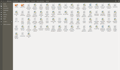And for the end of this section, let us connect our camera to one of our USB ports on Jetson Nano and check it with:

``ls /dev/video* ``

You should see a yellow line indicating that the device can see your camera: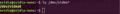## How to run Tensorflow Object Detection in Python

Since our preparations outside the docker are done, let us install our container and run it:

``cd docker pull nvcr.io/nvidia/l4t-ml:r32.5.0-py3 sudo docker run -it --rm --gpus all -e DISPLAY=:0 -v /tmp/.X11-unix:/tmp/.X11-unix -v /home/nvidia/Documents:/home/nvidia/Documents --device /dev/video0:/dev/video0 --network host nvcr.io/nvidia/l4t-ml:r32.5.0-py3 ``

Now, we should be inside our container. We need to create, write and run a python file, and in order to do that, we need to install nano and Tkinter:

``apt-get update apt-get install nano apt-get install python3-tk ``

After we got these installations, we need to create a python file inside Tensorflow folder, and write our code:

``cd ./home/nvidia/Documents/Tensorflow/models/research/object_detection/ touch Real_time_object_detection.py nano Real_time_object_detection.py ``

Now you should see an empty file like below: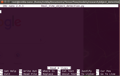Copy the below code and paste it with Ctrl + Shift + C to this empty file:

``import numpy as np import os import six.moves.urllib as urllib import sys import tarfile import tensorflow as tf import zipfile import tkinter from collections import defaultdict from io import StringIO from matplotlib import pyplot as plt from PIL import Image import cv2 cap = cv2.VideoCapture(0) sys.path.append("..") from utils import label_map_util from utils import visualization_utils as vis_util MODEL_NAME = 'ssd_mobilenet_v1_coco_11_06_2017' MODEL_FILE = MODEL_NAME + '.tar.gz' DOWNLOAD_BASE = 'http://download.tensorflow.org/models/object_detection/' # Path to frozen detection graph. This is the actual model that is used for the object detection. PATH_TO_CKPT = MODEL_NAME + '/frozen_inference_graph.pb' # List of the strings that is used to add correct label for each box. PATH_TO_LABELS = os.path.join('data', 'mscoco_label_map.pbtxt') NUM_CLASSES = 90 opener = urllib.request.URLopener() opener.retrieve(DOWNLOAD_BASE + MODEL_FILE, MODEL_FILE) tar_file = tarfile.open(MODEL_FILE) for file in tar_file.getmembers():    file_name = os.path.basename(file.name)    if 'frozen_inference_graph.pb' in file_name:        tar_file.extract(file, os.getcwd()) detection_graph = tf.Graph() with detection_graph.as_default():    od_graph_def = tf.GraphDef()    with tf.gfile.GFile(PATH_TO_CKPT, 'rb') as fid:        serialized_graph = fid.read()        od_graph_def.ParseFromString(serialized_graph)        tf.import_graph_def(od_graph_def, name='') label_map = label_map_util.load_labelmap(PATH_TO_LABELS) categories = label_map_util.convert_label_map_to_categories(label_map, max_num_classes=NUM_CLASSES, use_display_name=True) category_index = label_map_util.create_category_index(categories) with detection_graph.as_default():    with tf.Session(graph=detection_graph) as sess:        while True:            ret, image_np = cap.read()            image_np_expanded = np.expand_dims(image_np, axis=0)            image_tensor = detection_graph.get_tensor_by_name('image_tensor:0')            boxes = detection_graph.get_tensor_by_name('detection_boxes:0')            # Score is shown on the result image, together with class label.            scores = detection_graph.get_tensor_by_name('detection_scores:0')            classes = detection_graph.get_tensor_by_name('detection_classes:0')            num_detections = detection_graph.get_tensor_by_name('num_detections:0')            # Actual detection.            (boxes, scores, classes, num_detections) = sess.run(                [boxes, scores, classes, num_detections],               feed_dict={image_tensor: image_np_expanded})            # Visualization of the results of a detection.            vis_util.visualize_boxes_and_labels_on_image_array(                    image_np,                   np.squeeze(boxes),                   np.squeeze(classes).astype(np.int32),                   np.squeeze(scores),                   category_index,                   use_normalized_coordinates=True,                   line_thickness=8)            cv2.imshow('object detection', cv2.resize(image_np, (800,600)))            if cv2.waitKey(25) == ord('q'):                cv2.destroyAllWindows()        break ``

Then press Ctrl + X to quit, then press Y to save changes, and finally press ‘Enter’ to confirm the file’s name.

## How to detect objects in real time with Tensorflow

The name of the models we are using are ‘ssd_mobilenet_v1_coco_11_06_2017’. Now, we need to gain access to our camera from docker. Open a new terminal using Ctrl + Alt + T, and write the following:

``xhost + ``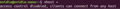We should see the following output from the terminal. Then let’s switch back to our first terminal and run our code, since we are ready:

``python3 Real_time_object_detection.py ``

Running our file may take a couple of minutes. You can see how it works below: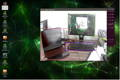Thank you for reading our blog post.